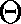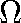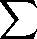# Lecture 2

Read Chapters 1 and 2 in the books. We will be discussing,andnotation.

# Example Continued

Last time, we saw that the number of array accesses done in selection sort on n items is n² + 3n - 4 . Now let's look at another sorting algorithm: merge sort. Merge sort is a recursive algorithm that splits the array into two subarrays, sorts each subarray, and then merges the two sorted arrays into a single sorted array. The base case of the recursion is when a subarray of size 1 (or 0) is reached; sorting a singleton or empty array is of course trivial.
Here is some C code that does merge sort. It assumes that two arrays, v1 and v2 have been allocated to be of size n/2; they will be used for the merging operation:
```void merge (float [], int, int, int);

/* sort the (sub)array v from start to end */

void merge_sort (float v[], int start, int end) {
int	middle;		/* the middle of the subarray */

/* no elements to sort */

if (start == end) return;

/* one element; already sorted! */

if (start == end - 1) return;

/* find the middle of the array, splitting it into two subarrays */

middle = (start + end) / 2;

/* sort the subarray from start..middle */

merge_sort (v, start, middle);

/* sort the subarray from middle..end */

merge_sort (v, middle, end);

/* merge the two sorted halves */

merge (v, start, middle, end);
}

/* merge the subarray v[start..middle] with v[middle..end], placing the
* result back into v.
*/
void merge (float v[], int start, int middle, int end) {
int	v1_n, v2_n, v1_index, v2_index, i;

/* number of elements in first subarray */

v1_n = middle - start;

/* number of elements in second subarray */

v2_n = end - middle;

/* fill v1 and v2 with the elements of the first and second
* subarrays, respectively
*/
for (i=0; i<v1_n; i++) v1[i] = v[start + i];
for (i=0; i<v2_n; i++) v2[i] = v[middle + i];

/* v1_index and v2_index will index into v1 and v2, respectively... */

v1_index = 0;
v2_index = 0;

/* ... as we pick elements from one or the other to place back
* into v
*/
for (i=0; (v1_index < v1_n) && (v2_index < v2_n); i++) {

/* current v1 element less than current v2 element? */

if (v1[v1_index] < v2[v2_index])

/* if so, this element belong as next in v */

v[start + i] = v1[v1_index++];
else

/* otherwise, the element from v2 belongs there */

v[start + i] = v2[v2_index++];
}

/* clean up; either v1 or v2 may have stuff left in it */

for (; v1_index < v1_n; i++) v[start + i] = v1[v1_index++];
for (; v2_index < v2_n; i++) v[start + i] = v2[v2_index++];
}
```
The function merge_sort is initially called with start equal to 0 and end equal to n, the number of floats in the array.

We need to come up with a function that expresses the number of array accesses done by merge sort. We'll call this function T(n). T will start out taking a recursive definition, just like the merge_sort function. At each recursive call of merge_sort, n in the definition of T will be the number of elements being sorted for this call, end - start.

Assume for convenience that n is a power of two. We can generalize this later (but we won't since we're lazy), but the analysis is easier this way. So each time we subdivide v into start..middle and middle..end, the subarrays are of equal size, each of size n/2. Thus in the function merge, v1_n = v2_n = n/2.

merge_sort is called recursively twice, each time with n half what it was before (e.g., start..middle is only half start..end). merge does a number of array accesses proportional to v1_n and v2_n (i.e., n/2). Now we can define T:

T(n) =
• 2T(n/2) + the time for merge with v1_n = v2_n = n/2, if n > 1,
• 0 otherwise.
The first two for loops in merge each do two array accesses n/2 times, for a total of 4n/2 = 2n accesses. The third loop is a little tricky; it may iterate anywhere from 0 to n times, leaving the next two loops to clean up. In the worst case, the third loop will execute n times. This is the worst case because it clearly does more accesses than the last two loops so will take longer. (We must often simplify the analysis of algorithms with this kind of worst-case analysis). In this case, four accesses per iteration are made: two in the comparison and two in whichever branch of the if statement is taken. So this loop does 4n array accesses. In the worst case, the last two loops are not executed at all, so we can ignore them. Thus the merge function does 6n accesses.

So now we have:

T(n) =
• 2T(n/2) + 6n, if n > 1,
• 0 otherwise.
We'd like to get a closed form for this if we can; this type of recurrence isn't very useful for comparing with other algorithms. There are standard tools for dealing with recurrence equations like this that involve complicated stuff we'd rather not get into now, but for now we'll just use simple algebra.

Let 2i = n (remember, we said n is a power of two; now we're just saying k is that power). Substituting and commuting, we get:

T(2i) =
• 6 · 2i + 2T(2i-1) if i > 0,
• 0 otherwise.
If we carry out the recursion, it looks like this:
6 · 2i + 2 (6 · 2i-1 + 2 (6 · 2i-2 + 2 (6 · 2i-3 + ... + 2 (6 · 21) + 0) ... )
Multiplying the twos through:
6 · 2i +2 · 6 · 2i-1 +4 · 6 · 2i-2 +8 · 6 · 2i-3 + ... + ? (6 · 21) + 0) ... )
We can rewrite this as:
2i = 2k[ 2k · 6 · 2i-k ] =
k = 0
2i = 2k
6[ 2k · 2i/2k ] =
k = 0
2i = 2k
6·2i1
k = 0
Substituting back, we get:
n = 2k
6n1 =
k = 0
k = log2 n
6n1 =
k = 0
6n(log2 n + 1) = 6n log2 n + 6n
And if we haven't had enough, we can remember that loga x = ln x / ln a. ln 2 is about .69314, dividing 6 by that gives about 8.6561, so the final result is
T(n) = 8.6561 n ln n + 6n
When we look at the orders of functions in terms of theta, omega, and "big-oh" notation, we'll see that the lower order terms and the constants are unimportant; n log n is the important term.

This function n log n grows much more slowly than the n2 term for selection sort as we saw in the last lecture, so merge sort is a much faster sort. This speed does come with a price, though: merge sort as presented above requires twice the memory since v1 and v2 must have sizes that sum to n.